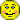Forum:

Need help evaluating logarithms
By Spiorad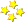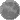On Wed Apr 03, 2013 06:49 AM

I cannot grasp this concept. My teacher is of absolutely no help. He doesn't have office hours, doesn't give extra credit, and zooms through things in class. I have emailed him several times and he is just completely useless. I have also been to tutoring for the last several weeks and it has been helping but now I have a test coming up on Tuesday and I have to work when the tutoring lab is open.

Basically tonight's homework is completely stumping me. I have no idea what the heck to do. I have no idea where to even start!

I took a picture of the first 3 problems. The directions say: Use properties of logarithms to find the exact value of each expression. Do not use a calculator.

I am hoping that if someone can help me understand these three I can maybe figure out how to do the rest. (Though they are different problems and you are asked to do different things for each section)

For the first one:
Is this where you have to rewrite it as -13 log base 2 (2)?
Or is this where you have to figure out how many 2's and times something else gives -13?

Or is it:
2^X=-13
log2^x=log-13 (or was am I supposed to take the log of both sides?)
xlog2=log-13
and... then I have no idea. At all.

See? I have absolutely NO idea what the heck I am doing. I am just getting so beyond frustrated with this. It is 12:47am an I am no closer to getting this ish done then I was a few hours ago. :/

Lets just start with these three and if someone is able to explain to me this stuff in a way I can understand I would really appreciate it. And if you have time for me to ask some more questions after that would be great.
x= (log-13/log2)

### 3 Replies to Need help evaluating logarithms

re: Need help evaluating logarithms
By Lauranne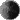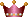On Wed Apr 03, 2013 12:39 PM
Hello.

I usually find that wikipedia is of great help for basic math work.

As a start, let me just give you the links to the pages where you'll find the identities rules for the logarithm:
en.wikipedia.org . . . (rules + example - see table in section Logarithmic identities)
and for the special case of the natural logarithm: en.wikipedia.org . . .

The first link should allow you to answer problems 1 and 3. The second link is for problem 2. You don't have to work with any x's. It's "simple" mathematics, with all quantities known.

I'll come back regularly to this thread so don't hesitate to post the answer you found and your questions.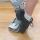re: Need help evaluating logarithms
By Bril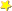On Wed Apr 03, 2013 04:41 PM
For question one, since the base and the log number are the same, it is the inverse rule:
log base a (a^x) = x : the a's here match leaving the x
So log base 2 (2^-13) : the 2's here match leaving the -13
(Sorry if it is hard to understand, I can't paste math into this text box)
For the second question, since ln=log base e, it is the same rule as the first question but in reverse.
a^(log base a (x)) = x : the a's here match leaving the x
e^(log base e (8)) : the e's here match leaving the 8
For the third question, it is the same as question one, once you factor it into two pieces
5^(log base 5 (6)+ log base 5 (7))
using the exponent rule becomes:
5^log base 5 (6) (one piece) * 5^log base 5 (7) (second piece)
This reduces as in question one to 6*7

Feel free to ask me any math questions you might have or if I am not clear anywhere here. I am always happy to help out with math.